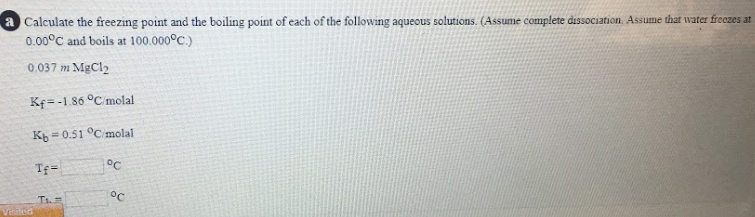# a) Calculate the freezing point and the boiling point of each of the following aqueous solutions. (Assume complete dissociation. Assume that water freezes at 0.00 degrees C and boils at 100.000 degrees C) Kf = -1.86 degrees C/molal Kb = 0.51 degrees C/molal Tf = ___ degrees C Tb = ___ degrees C